In :
from skimage.io import imread
from skimage.color import rgb2gray
import matplotlib.pyplot as plt

plt.figure(figsize=(10,10))
plt.axis("off")
plt.imshow(img, cmap="gray")
plt.show()

<Figure size 1000x1000 with 1 Axes>

### Find the Horizontal projection profile and find the window where line segment can be created.¶

One of the common ways of finding the line-height of a document is by analyzing its Horizontal projection profile. Horizontal projection profile (HPP) is the array of sum or rows of a two dimentional image. Where there are more white spaces we see more peaks. These peaks give us an idea of where the segmentation between two lines can be done.

In :
from skimage.filters import sobel
import numpy as np

def horizontal_projections(sobel_image):
return np.sum(sobel_image, axis=1)

sobel_image = sobel(img)
hpp = horizontal_projections(sobel_image)
plt.plot(hpp)
plt.show()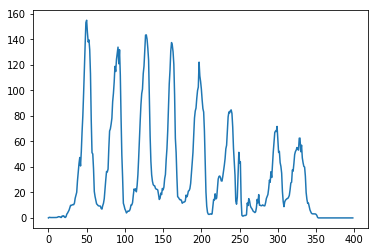As you can see, where there were more white spaces there are peaks in the graph. We will use this information further to locate the regions where we can find the seperation line.

In :
#find the midway where we can make a threshold and extract the peaks regions
#divider parameter value is used to threshold the peak values from non peak values.
def find_peak_regions(hpp, divider=2):
threshold = (np.max(hpp)-np.min(hpp))/divider
peaks = []
peaks_index = []
for i, hppv in enumerate(hpp):
if hppv < threshold:
peaks.append([i, hppv])
return peaks

peaks = find_peak_regions(hpp)

peaks_index = np.array(peaks)[:,0].astype(int)

segmented_img = np.copy(img)
r,c = segmented_img.shape
for ri in range(r):
if ri in peaks_index:
segmented_img[ri, :] = 0

plt.figure(figsize=(20,20))
plt.imshow(segmented_img, cmap="gray")
plt.show()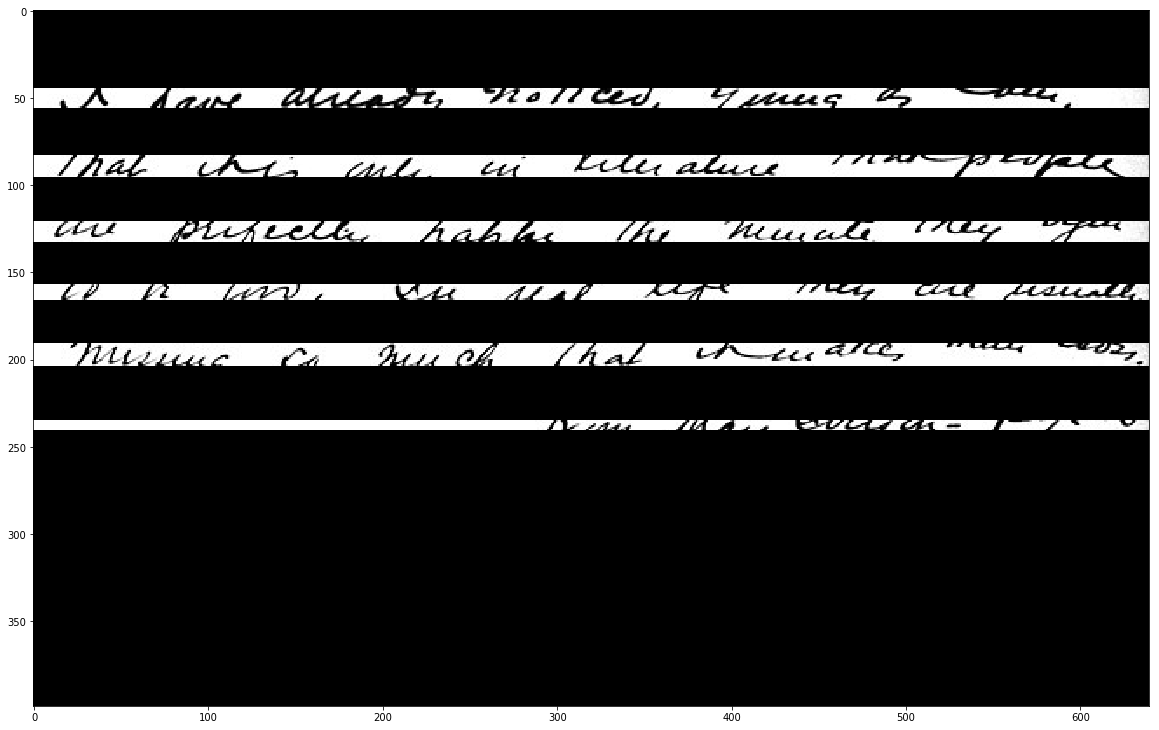The above black regions indicate where we would need to run our path planning algorithm for line segmentation.

In :
#group the peaks into walking windows
def get_hpp_walking_regions(peaks_index):
hpp_clusters = []
cluster = []
for index, value in enumerate(peaks_index):
cluster.append(value)

if index < len(peaks_index)-1 and peaks_index[index+1] - value > 1:
hpp_clusters.append(cluster)
cluster = []

#get the last cluster
if index == len(peaks_index)-1:
hpp_clusters.append(cluster)
cluster = []

return hpp_clusters

hpp_clusters = get_hpp_walking_regions(peaks_index)

In :
#a star path planning algorithm
from heapq import *

def heuristic(a, b):
return (b - a) ** 2 + (b - a) ** 2

def astar(array, start, goal):

neighbors = [(0,1),(0,-1),(1,0),(-1,0),(1,1),(1,-1),(-1,1),(-1,-1)]
close_set = set()
came_from = {}
gscore = {start:0}
fscore = {start:heuristic(start, goal)}
oheap = []

heappush(oheap, (fscore[start], start))

while oheap:

current = heappop(oheap)

if current == goal:
data = []
while current in came_from:
data.append(current)
current = came_from[current]
return data

for i, j in neighbors:
neighbor = current + i, current + j
tentative_g_score = gscore[current] + heuristic(current, neighbor)
if 0 <= neighbor < array.shape:
if 0 <= neighbor < array.shape:
if array[neighbor][neighbor] == 1:
continue
else:
# array bound y walls
continue
else:
# array bound x walls
continue

if neighbor in close_set and tentative_g_score >= gscore.get(neighbor, 0):
continue

if  tentative_g_score < gscore.get(neighbor, 0) or neighbor not in [ifor i in oheap]:
came_from[neighbor] = current
gscore[neighbor] = tentative_g_score
fscore[neighbor] = tentative_g_score + heuristic(neighbor, goal)
heappush(oheap, (fscore[neighbor], neighbor))

return []

In :
#Scan the paths to see if there are any blockers.
from skimage.filters import threshold_otsu
from skimage.util import invert

def get_binary(img):
mean = np.mean(img)
if mean == 0.0 or mean == 1.0:
return img

thresh = threshold_otsu(img)
binary = img <= thresh
binary = binary*1
return binary

def path_exists(window_image):
#very basic check first then proceed to A* check
if 0 in horizontal_projections(window_image):
return True

path = np.array(astar(world_map, (int(world_map.shape/2), 0), (int(world_map.shape/2), world_map.shape)))
if len(path) > 0:
return True

return False

needtobreak = False

for col in range(nmap.shape):
start = col
end = col+20
if end > nmap.shape-1:
end = nmap.shape-1
needtobreak = True

if path_exists(nmap[:, start:end]) == False:

if needtobreak == True:
break

if index == size-1 and len(road_blocks_cluster) > 0:

binary_image = get_binary(img)

for cluster_of_interest in hpp_clusters:
nmap = binary_image[cluster_of_interest:cluster_of_interest[len(cluster_of_interest)-1],:]
#create the doorways

In :
#now that everything is cleaner, its time to segment all the lines using the A* algorithm
line_segments = []
for i, cluster_of_interest in enumerate(hpp_clusters):
nmap = binary_image[cluster_of_interest:cluster_of_interest[len(cluster_of_interest)-1],:]
path = np.array(astar(nmap, (int(nmap.shape/2), 0), (int(nmap.shape/2),nmap.shape-1)))
offset_from_top = cluster_of_interest
path[:,0] += offset_from_top
line_segments.append(path)

In :
cluster_of_interest = hpp_clusters
offset_from_top = cluster_of_interest
nmap = binary_image[cluster_of_interest:cluster_of_interest[len(cluster_of_interest)-1],:]
plt.figure(figsize=(20,20))
plt.imshow(invert(nmap), cmap="gray")

path = np.array(astar(nmap, (int(nmap.shape/2), 0), (int(nmap.shape/2),nmap.shape-1)))
plt.plot(path[:,1], path[:,0])

Out:
[<matplotlib.lines.Line2D at 0x109664898>]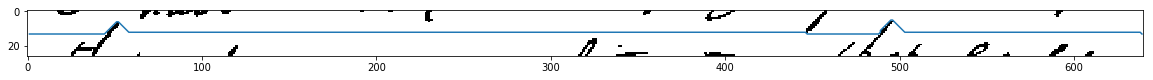In :
offset_from_top = cluster_of_interest
fig, ax = plt.subplots(figsize=(20,10), ncols=2)
for path in line_segments:
ax.plot((path[:,1]), path[:,0])
ax.axis("off")
ax.axis("off")
ax.imshow(img, cmap="gray")
ax.imshow(img, cmap="gray")

Out:
<matplotlib.image.AxesImage at 0x10963a588>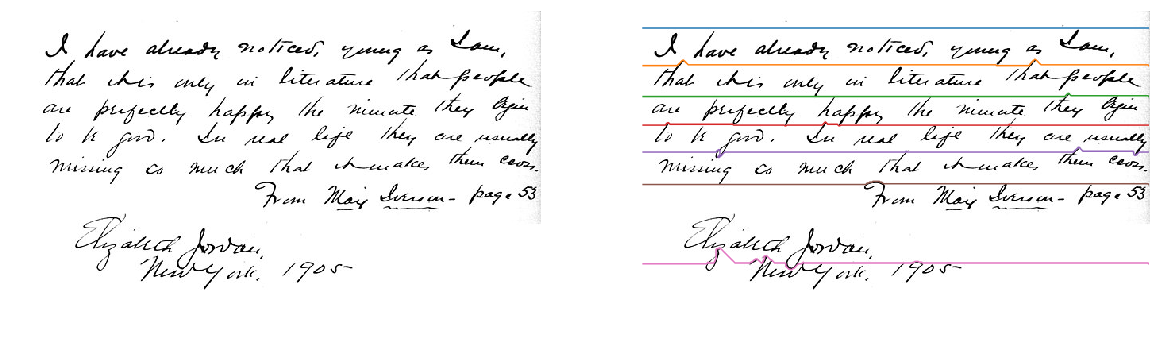In :
## add an extra line to the line segments array which represents the last bottom row on the image
last_bottom_row = np.flip(np.column_stack(((np.ones((img.shape,))*img.shape), np.arange(img.shape))).astype(int), axis=0)
line_segments.append(last_bottom_row)


### Lets divide the image now by the line segments passing through the image¶

In :
line_images = []
def extract_line_from_image(image, lower_line, upper_line):
lower_boundary = np.min(lower_line[:, 0])
upper_boundary = np.min(upper_line[:, 0])
img_copy = np.copy(image)
r, c = img_copy.shape
for index in range(c-1):
img_copy[0:lower_line[index, 0], index] = 255
img_copy[upper_line[index, 0]:r, index] = 255

return img_copy[lower_boundary:upper_boundary, :]

line_count = len(line_segments)
fig, ax = plt.subplots(figsize=(10,10), nrows=line_count-1)
for line_index in range(line_count-1):
line_image = extract_line_from_image(img, line_segments[line_index], line_segments[line_index+1])
line_images.append(line_image)
ax[line_index].imshow(line_image, cmap="gray")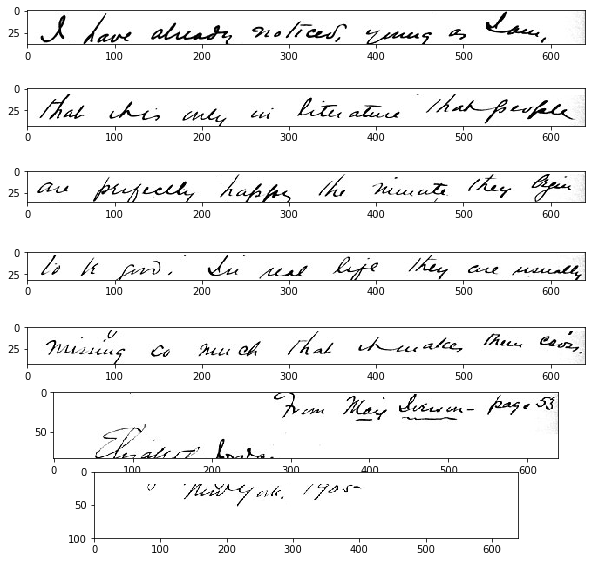### now that I have the lines, I can now divide these lines into words.¶

In :
from skimage.filters import threshold_otsu

#binarize the image, guassian blur will remove any noise in the image
first_line = line_images
thresh = threshold_otsu(first_line)
binary = first_line > thresh

# find the vertical projection by adding up the values of all pixels along rows
vertical_projection = np.sum(binary, axis=0)

# plot the vertical projects
fig, ax = plt.subplots(nrows=2, figsize=(20,10))
plt.xlim(0, first_line.shape)
ax.imshow(binary, cmap="gray")
ax.plot(vertical_projection)

Out:
[<matplotlib.lines.Line2D at 0x109cd7748>]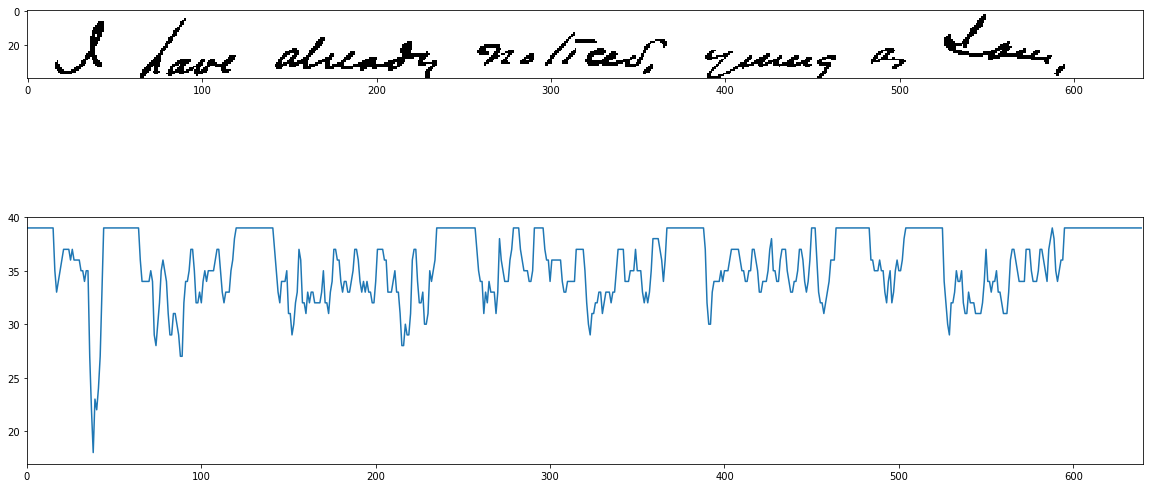In :
height = first_line.shape

## we will go through the vertical projections and
## find the sequence of consecutive white spaces in the image
whitespace_lengths = []
whitespace = 0
for vp in vertical_projection:
if vp == height:
whitespace = whitespace + 1
elif vp != height:
if whitespace != 0:
whitespace_lengths.append(whitespace)
whitespace = 0 # reset whitepsace counter.

print("whitespaces:", whitespace_lengths)
avg_white_space_length = np.mean(whitespace_lengths)
print("average whitespace lenght:", avg_white_space_length)

whitespaces: [16, 21, 22, 23, 4, 6, 22, 3, 20, 22, 1]
average whitespace lenght: 14.545454545454545

In :
## find index of whitespaces which are actually long spaces using the avg_white_space_length
whitespace_length = 0
divider_indexes = []
for index, vp in enumerate(vertical_projection):
if vp == height:
whitespace_length = whitespace_length + 1
elif vp != height:
if whitespace_length != 0 and whitespace_length > avg_white_space_length:
divider_indexes.append(index-int(whitespace_length/2))
whitespace_length = 0 # reset it

print(divider_indexes)

[8, 55, 131, 247, 373, 473, 515]

In :
# lets create the block of words from divider_indexes
divider_indexes = np.array(divider_indexes)
dividers = np.column_stack((divider_indexes[:-1],divider_indexes[1:]))

In :
# now plot the findings
fig, ax = plt.subplots(nrows=len(dividers), figsize=(5,10))
for index, window in enumerate(dividers):
ax[index].axis("off")
ax[index].imshow(first_line[:,window:window], cmap="gray")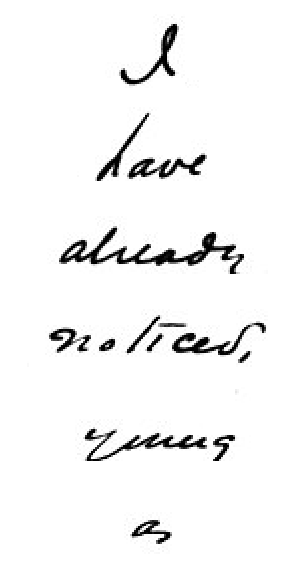In [ ]: